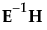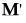In the following, E is the residual cross product matrix and H is the model cross product matrix. Diagonal elements of E are the residual sums of squares for each variable. Diagonal elements of H are the sums of squares for the model for each variable. In the discriminant analysis literature, E is often called W, where W stands for within.
Test statistics in the multivariate results tables are functions of the eigenvalues λ of. The following list describes the computation of each test statistic.
Note: After specification of a response design, the initial E and H matrices are premultiplied byand postmultiplied by M.
 • Wilks’ Lambda
 • Pillai’s Trace
 • Hotelling-Lawley Trace
 • Roy’s Max Root
E and H are defined as follows:
where b is the estimated vector for the model coefficients and A- denotes the generalized inverse of a matrix A.
The whole model L is a column of zeros (for the intercept) concatenated with an identity matrix having the number of rows and columns equal to the number of parameters in the model. L matrices for effects are subsets of rows from the whole model L matrix.

Help created on 9/19/2017